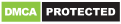# Calculator

Genres
Version
-
Developer
-
Requires
-
Updated
-Calculator provides simple and advanced mathematical functions in a beautifully designed app.

• Perform basic calculations such as addition, subtraction, multiplication, and division
• Do scientific operations such as trigonometric, logarithmic, and exponential functions
• Calculator now displays decimal results and symbolic results for fractions or certain advanced functions. For example, 10 ÷ 12 will display both 0.83333… and ⅚.

Recommended for you

You may also like